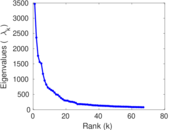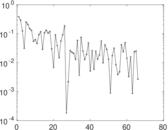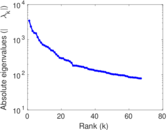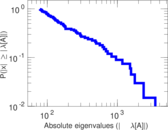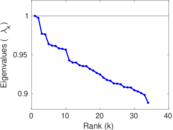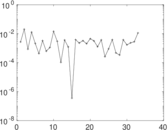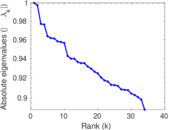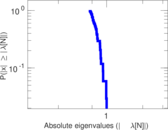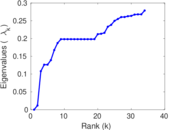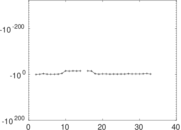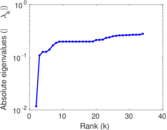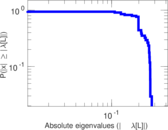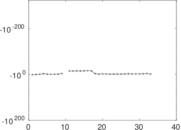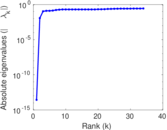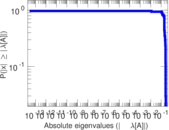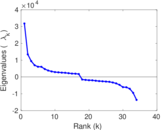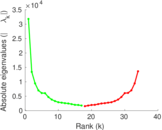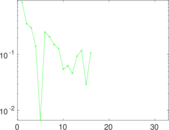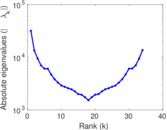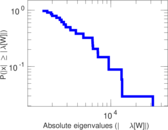# Wikivoyage edits (it)

This is the bipartite edit network of the Italian Wikivoyage. It contains users and pages from the Italian Wikivoyage, connected by edit events. Each edge represents an edit. The dataset includes the timestamp of each edit.

 Code `vit` Internal name `edit-itwikivoyage` Name Wikivoyage edits (it) Data source http://dumps.wikimedia.org/ AvailabilityDataset is available for download Consistency checkDataset passed all tests Category Authorship network Dataset timestamp 2017-10-20 Node meaning User, article Edge meaning Edit Network formatBipartite, undirected Edge typeUnweighted, multiple edges Temporal dataEdges are annotated with timestamps

## Statistics

 Size n = 31,501 Left size n1 = 2,538 Right size n2 = 28,963 Volume m = 419,474 Unique edge count m̿ = 80,998 Wedge count s = 233,719,503 Claw count z = 947,377,766,551 Cross count x = 3,370,127,980,678,628 Square count q = 121,744,148 4-Tour count T4 = 1,909,097,784 Maximum degree dmax = 105,298 Maximum left degree d1max = 105,298 Maximum right degree d2max = 4,645 Average degree d = 26.632 4 Average left degree d1 = 165.277 Average right degree d2 = 14.483 1 Fill p = 0.001 101 89 Average edge multiplicity m̃ = 5.178 82 Size of LCC N = 30,898 Diameter δ = 11 50-Percentile effective diameter δ0.5 = 3.067 63 90-Percentile effective diameter δ0.9 = 3.960 59 Median distance δM = 4 Mean distance δm = 3.300 61 Gini coefficient G = 0.901 896 Balanced inequality ratio P = 0.112 204 Left balanced inequality ratio P1 = 0.042 612 9 Right balanced inequality ratio P2 = 0.146 944 Relative edge distribution entropy Her = 0.718 827 Power law exponent γ = 2.767 12 Tail power law exponent γt = 2.001 00 Tail power law exponent with p γ3 = 2.001 00 p-value p = 0.000 00 Left tail power law exponent with p γ3,1 = 1.831 00 Left p-value p1 = 0.000 00 Right tail power law exponent with p γ3,2 = 4.991 00 Right p-value p2 = 0.060 000 0 Degree assortativity ρ = −0.254 768 Degree assortativity p-value pρ = 0.000 00 Spectral norm α = 3,468.77 Algebraic connectivity a = 0.011 697 0 Spectral separation |λ1[A] / λ2[A]| = 1.467 30 Controllability C = 27,715 Relative controllability Cr = 0.888 501

## Plots

### Fruchterman–Reingold graph drawing### Degree distribution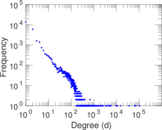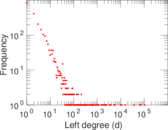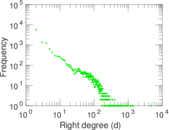### Cumulative degree distribution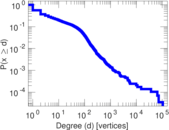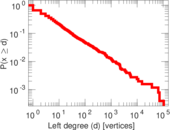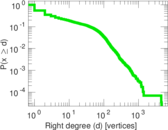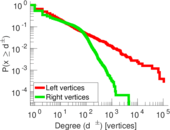### Lorenz curve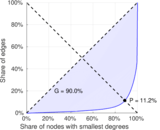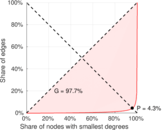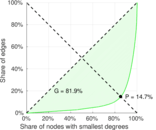### Spectral distribution of the adjacency matrix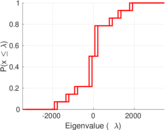### Spectral distribution of the normalized adjacency matrix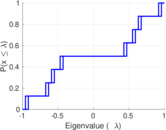### Spectral distribution of the Laplacian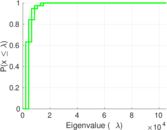### Spectral graph drawing based on the adjacency matrix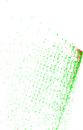### Spectral graph drawing based on the Laplacian### Spectral graph drawing based on the normalized adjacency matrix### Degree assortativity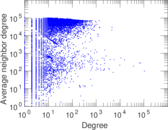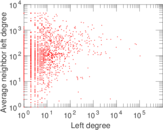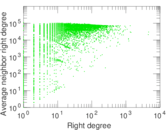### Zipf plot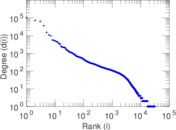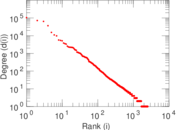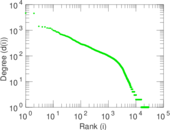### Hop distribution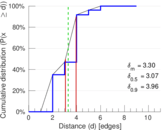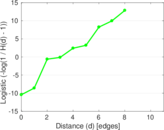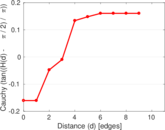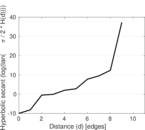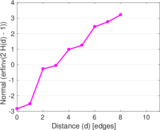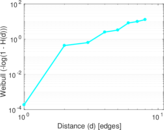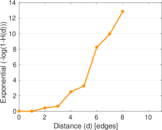### Double Laplacian graph drawing### Delaunay graph drawing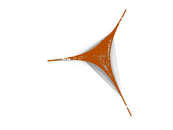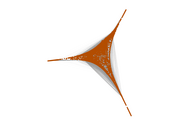### Edge weight/multiplicity distribution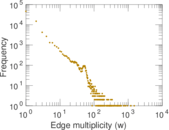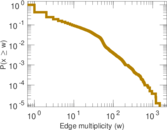### Temporal distribution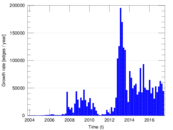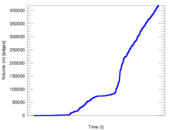### Temporal hop distribution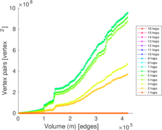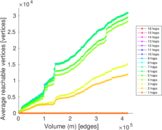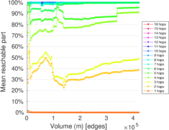### Diameter/density evolution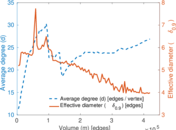### Matrix decompositions plots